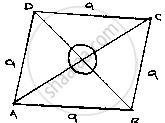# Find the Area of a Rhombus Whose Perimeter is 80 M and One of Whose Diagonal is 24 M. - Mathematics

Find the area of a rhombus whose perimeter is 80 m and one of whose diagonal is 24 m.

#### Solution

Given that,
Perimeter of rhombus = 80m
Perimeter of rhombus = 4 × side⇒ 4a = 80
⇒ a = 20m
Let AC = 24 m

∴ OA =1/2AC=1/2xx24=12m

In ΔAOB

OB^2=AB^2-OA^2

⇒OB=sqrt(20^2-12^2)

sqrt(400-144)

sqrt(256)=16m

Also BO = OD

[Diagonal of rhombus bisect each other at 90°]

∴ BD = 20B = 2 ×16 = 32 m

∴Area of rhombus = 1/2×32×24=384𝑚^2

[∵Area of rhombus = 12×𝐵𝐷×𝐴𝐶]

Concept: Application of Heron’s Formula in Finding Areas of Quadrilaterals
Is there an error in this question or solution?

#### APPEARS IN

RD Sharma Mathematics for Class 9
Chapter 17 Heron’s Formula
Exercise 17.2 | Q 12 | Page 20

Share